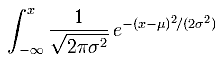# Modern Blackjack nth Edition

#### Calculation of Area Under the Standard Normal Curve

###### Rate this Entry
by , 04-25-2012 at 03:53 AM (9901 Views)
On occasion I will post code to the blog as an extension of the Sim Secrets chapter of my book. These posts will generally only be of interest to programmers.

Page 91 in Theory of Blackjack and page 147 in Blackjack Attack have tables used to determine areas under sections of the standard normal curve. These tables can be used to determine the probability of some events. For example, CVCX uses this to determine the probability of your actual results in a casino.

The formula for calculating the area to the right of a point (x) on the curve is 1 minus:This is the cumulative distribution function (image pieced together from WP). In Excel, it is calculated using Norm.Dist. But, it’s a royal pain to estimate in code. So, I have included the code I use below, taken from a long forgotten French site years back and adapted for the problem at hand:

Function normp(z As Double) As Double
Dim zabs As Double, q As Double, pdf As Double, p As Double, expntl As Double
‘Normal distribution probabilities accurate to 1.e-15.
‘Z = no. of standard deviations from the mean.
‘P, Q = probabilities to the left & right of Z. P + Q = 1.
‘PDF = the probability density.
‘Based upon algorithm 5666 for the error function, from:
‘Hart, J.F. et al, ‘Computer Approximations’, Wiley 1968
Const p0 = 220.206867912376
Const p1 = 221.213596169931
Const p2 = 112.079291497871
Const p3 = 33.912866078383
Const p4 = 6.37396220353165
Const p5 = 0.700383064443688
Const p6 = 3.52624965998911E-02
Const q0 = 440.413735824752
Const q1 = 793.826512519948
Const q2 = 637.333633378831
Const q3 = 296.564248779674
Const q4 = 86.7807322029461
Const q5 = 16.064177579207
Const q6 = 1.75566716318264
Const q7 = 8.83883476483185E-02
Const cutoff = 7.071
Const root2pi = 2.506628274631
GoSub normpcalculs
Exit Function
normpcalculs:
zabs = Abs(z)
‘***** |Z| > 37.
If (zabs > 37#) Then
pdf = 0#
If (z > 0#) Then
p = 1#
normp = p
q = 0#
Else
p = 0#
normp = p
q = 1#
End If
Return
End If
‘***** |Z| <= 37.
expntl = Exp(-0.5 * zabs ^ 2)
pdf = expntl / root2pi
‘***** |Z| < CUTOFF = 10/sqrt(2).
If (zabs < cutoff) Then
p = expntl * ((((((p6 * zabs + p5) * zabs + p4) * zabs + p3) * zabs + p2) * zabs + p1) * zabs + p0) / (((((((q7 * zabs + q6) * zabs + q5) * zabs + q4) * zabs + q3) * zabs + q2) * zabs + q1) * zabs + q0)
normp = p
Else ‘|Z| >= CUTOFF.
‘p = pdf / (zabs + 1# / (zabs + 2# / (zabs + 3# / (zabs + 4# / (zabs + 0.65)))))
p = (zabs + 0.65)
p = (zabs + 4# / p)
p = pdf / (zabs + 1# / (zabs + 2# / (zabs + 3# / p)))
normp = p
End If
If (z < 0#) Then
q = 1# – p
Else
q = p
p = 1# – q
normp = p
End If
Return
End Function
Categories
Code